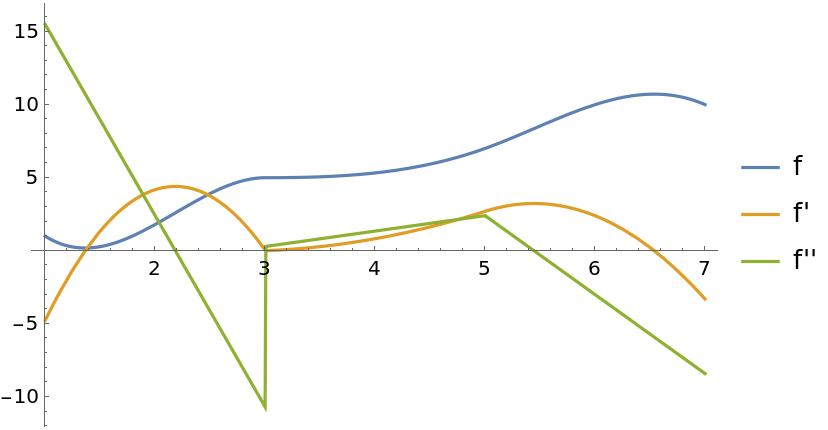#Function Repository Resource:

# CubicSplineInterpolation

Interpolate data with a cubic spline

Contributed by: Jan Mangaldan
 ResourceFunction["CubicSplineInterpolation"][{f1,f2,…}] constructs a cubic spline interpolation of the function values fi, assumed to correspond to x values 1, 2, …. ResourceFunction["CubicSplineInterpolation"][{{x1,f1},{x2,f2},…}] constructs a cubic spline interpolation of the function values fi corresponding to x values xi. ResourceFunction["CubicSplineInterpolation"][data,cond] uses conditions for the left and right endpoints specified by cond.

## Details

ResourceFunction["CubicSplineInterpolation"] returns an InterpolatingFunction object, which can be used like any other pure function.
The interpolation function returned by ResourceFunction["CubicSplineInterpolation"][data] is set up so as to agree with data at every point explicitly specified in data.
ResourceFunction["CubicSplineInterpolation"] yields an interpolant with continuous first and second derivatives.
The function values fi are expected to be real or complex numbers.
The function arguments xi must be real numbers.
ResourceFunction["CubicSplineInterpolation"][data] generates an InterpolatingFunction object that returns values with the same precision as those in data.
The following endpoint conditions can be specified for cond:
 "NotAKnot" not-a-knot end condition "Natural" natural end condition (zero second derivative) "Clamped" set derivative at endpoint equal to the derivative of the polynomial passing through first (or last) four points {"Clamped",d} set derivative at endpoint equal to d {"Clamped",InterpolationOrder→m} set derivative at endpoint equal to the derivative of the polynomial passing through first (or last) m+1 points "Second" set second derivative at endpoint equal to 0 {"Second",d} set second derivative at endpoint equal to d {"Second",InterpolationOrder→m} set second derivative at endpoint equal to the second derivative of the polynomial passing through first (or last) m+1 points "Periodic" periodic
ResourceFunction["CubicSplineInterpolation"][data] is equivalent to ResourceFunction["CubicSplineInterpolation"][data,"Periodic"] if the left and right endpoints of data have the same ordinate, and ResourceFunction["CubicSplineInterpolation"][data,"NotAKnot"] otherwise.
ResourceFunction["CubicSplineInterpolation"][data,{cond1,cond2}] uses cond1 for the left endpoint condition and cond2 for the right endpoint condition.

## Examples

### Basic Examples (3)

Construct an approximate function that interpolates the data:

 In:=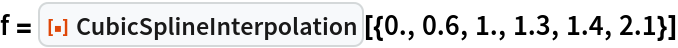Out=Apply the function to find interpolated values:

 In:=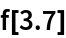Out=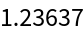Plot the interpolation function along with the original data:

 In:=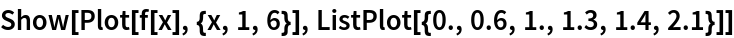Out=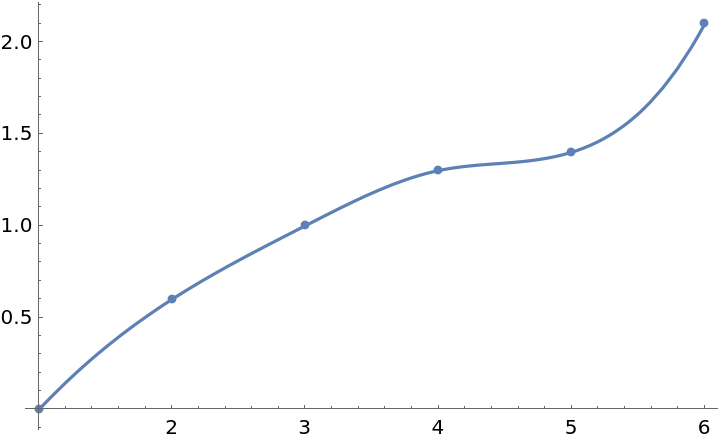### Scope (5)

Interpolate between points at arbitrary x values:

 In:=Out=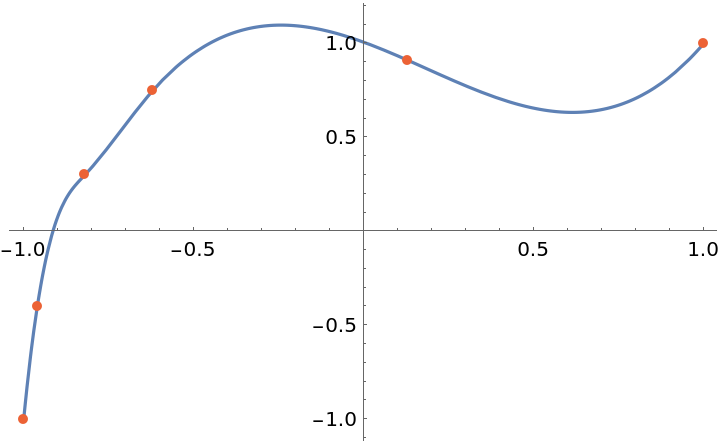Plot a natural cubic spline:

 In:=Out=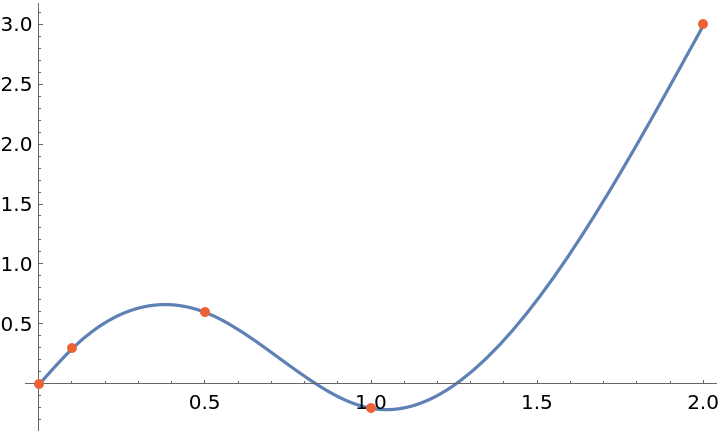Make a cubic spline with different left and right endpoint conditions:

 In:=Out=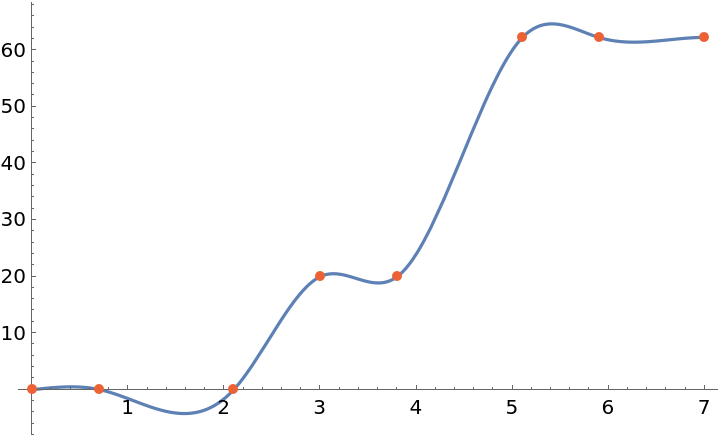Check that the second derivative at the left endpoint is reproduced:

 In:=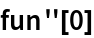Out=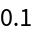Form an interpolation from data given as a TimeSeries:

 In:=Out=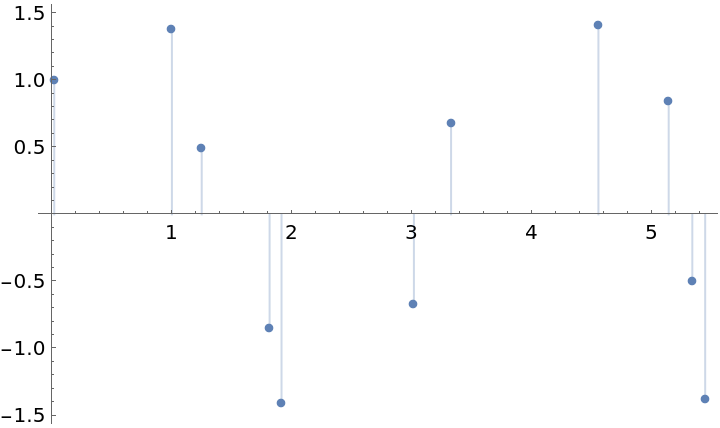In:=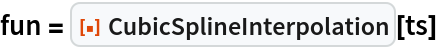Out=In:=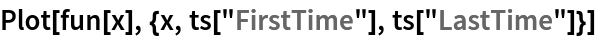Out=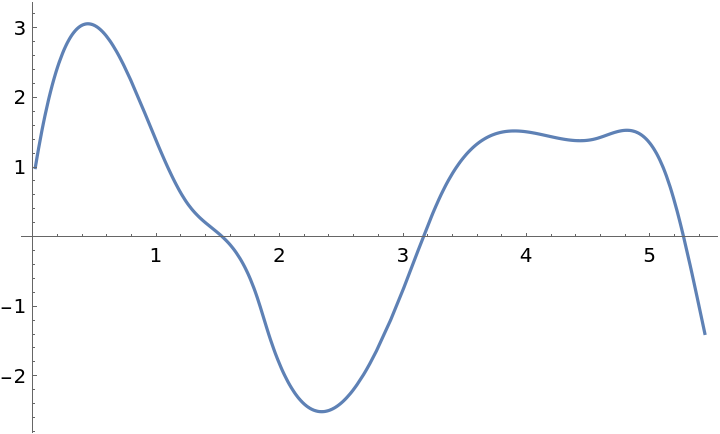A periodic cubic spline:

 In:=Out=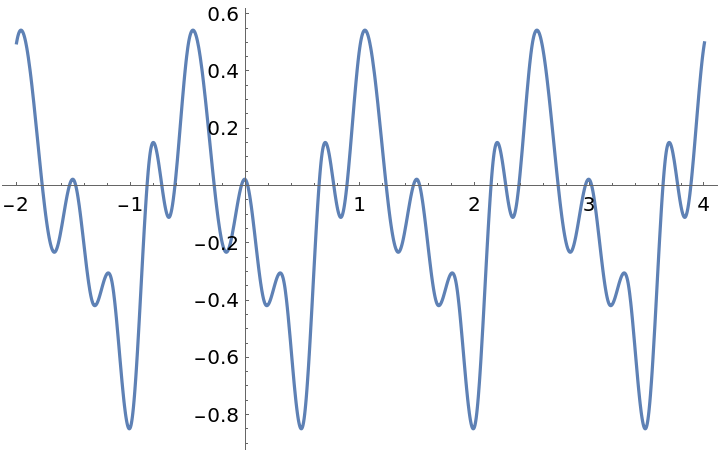### Properties and Relations (1)

The interpolant returned by CubicMonotonicInterpolation is continuous up to its second derivative:

 In:=Out=